# Variational Bayesian sparse additive matrix factorization

## Abstract

Principal component analysis (PCA) approximates a data matrix with a low-rank one by imposing sparsity on its singular values. Its robust variant can cope with spiky noise by introducing an element-wise sparse term. In this paper, we extend such sparse matrix learning methods, and propose a novel framework called sparse additive matrix factorization (SAMF). SAMF systematically induces various types of sparsity by a Bayesian regularization effect, called model-induced regularization. Although group LASSO also allows us to design arbitrary types of sparsity on a matrix, SAMF, which is based on the Bayesian framework, provides inference without any requirement for manual parameter tuning. We propose an efficient iterative algorithm called the mean update (MU) for the variational Bayesian approximation to SAMF, which gives the global optimal solution for a large subset of parameters in each step. We demonstrate the usefulness of our method on benchmark datasets and a foreground/background video separation problem.

## Introduction

Principal component analysis (PCA) (Hotelling 1933) is a classical method for obtaining low-dimensional expression of data. PCA can be regarded as approximating a data matrix with a low-rank one by imposing sparsity on its singular values. A robust variant of PCA further copes with sparse spiky noise included in observations (Candès et al. 2011; Ding et al. 2011; Babacan et al. 2012).

In this paper, we extend the idea of robust PCA, and propose a more general framework called sparse additive matrix factorization (SAMF). The proposed SAMF can handle various types of sparse noise such as row-wise and column-wise sparsity, in addition to element-wise sparsity (spiky noise) and low-rank sparsity (low-dimensional expression); furthermore, their arbitrary additive combination is also allowed. In the context of robust PCA, row-wise and column-wise sparsity can capture noise observed when some sensors are broken and their outputs are always unreliable, or some accident disturbs all sensor outputs at a time.

Flexibility of SAMF in sparsity design allows us to incorporate side information more efficiently. We show such an example in foreground/background video separation, where sparsity is induced based on image segmentation. Although group LASSO (Yuan and Lin 2006; Raman et al. 2009) also allows arbitrary sparsity design on matrix entries, SAMF, which is based on the Bayesian framework, enables us to estimate all unknowns from observations, and allows us to enjoy inference without manual parameter tuning.

Technically, our approach induces sparsity by the so-called model-induced regularization (MIR) (Nakajima and Sugiyama 2011). MIR is an implicit regularization property of the Bayesian approach, which is based on one-to-many (i.e., redundant) mapping of parameters and outcomes (Watanabe 2009). In the case of matrix factorization, an observed matrix is decomposed into two redundant matrices, which was shown to induce sparsity in the singular values under the variational Bayesian approximation (Nakajima and Sugiyama 2011).

We show that MIR in SAMF can be interpreted as automatic relevance determination (ARD) (Neal 1996), which is a popular Bayesian approach to inducing sparsity. Nevertheless, we argue that the MIR formulation is more preferable since it allows us to derive a practically useful algorithm called the mean update (MU) from a recent theoretical result (Nakajima et al. 2013): The MU algorithm is based on the variational Bayesian approximation, and gives the global optimal solution for a large subset of parameters in each step. Through experiments, we show that the MU algorithm compares favorably with a standard iterative algorithm for variational Bayesian inference.

## Formulation

In this section, we formulate the sparse additive matrix factorization (SAMF) model.

### Examples of factorization

In standard MF, an observed matrix $$V \in\mathbb{R}^{L \times M}$$ is modeled by a low rank target matrix $$U \in\mathbb{R}^{L \times M}$$ contaminated with a random noise matrix $$\mathcal{E} \in\mathbb{R}^{L \times M}$$.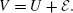Then the target matrix U is decomposed into the product of two matrices $$A \in\mathbb{R}^{M \times H}$$ and $$B \in\mathbb {R}^{L \times H}$$: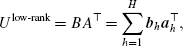(1)

where ⊤ denotes the transpose of a matrix or vector. Throughout the paper, we denote a column vector of a matrix by a bold small letter, and a row vector by a bold small letter with a tilde: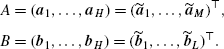The last equation in Eq. (1) implies that the plain matrix product (i.e., BA ) is the sum of rank-1 components. It was elucidated that this product induces an implicit regularization effect called model-induced regularization (MIR), and a low-rank (singular-component-wise sparse) solution is produced under the variational Bayesian approximation (Nakajima and Sugiyama 2011).

Let us consider other types of factorization: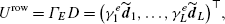(2)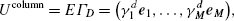(3)

where $$\varGamma_{D} = \textrm {diag}(\gamma^{d}_{1}, \ldots, \gamma^{d}_{M}) \in\mathbb {R}^{M \times M}$$ and $$\varGamma_{E} = \textrm {diag}(\gamma^{e}_{1}, \ldots, \gamma^{e}_{L}) \in\mathbb {R}^{L \times L}$$ are diagonal matrices, and $$D, E \in\mathbb{R}^{L \times M}$$. These examples are also matrix products, but one of the factors is restricted to be diagonal. Because of this diagonal constraint, the l-th diagonal entry $$\gamma_{l}^{e}$$ in Γ E is shared by all the entries in the l-th row of U row as a common factor. Similarly, the m-th diagonal entry $$\gamma_{m}^{d}$$ in Γ D is shared by all the entries in the m-th column of U column.

Another example is the Hadamard (or element-wise) product: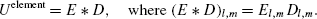(4)

In this factorization form, no entry in E and D is shared by more than one entry in U element.

In fact, the forms (2)–(4) of factorization induce different types of sparsity, through the MIR mechanism. In Sect. 2.2, they will be derived as a row-wise, a column-wise, and an element-wise sparsity inducing terms, respectively, within a unified framework.

### A general expression of factorization

Our general expression consists of partitioning, rearrangement, and factorization. The following is the form of a sparse matrix factorization (SMF) term: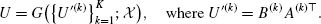(5)

Here, $$\{A^{(k)}, B^{(k)} \}_{k=1}^{K}$$ are parameters to be estimated, and $$G( \cdot; \mathcal{X}): \mathbb{R}^{\prod_{k=1}^{K} (L'^{(k)} \times M'^{(k)})} \mapsto\mathbb{R}^{L \times M}$$ is a designed function associated with an index mapping parameter $$\mathcal{X}$$, which will be explained shortly.

Figure 1 shows how to construct an SMF term. First, we partition the entries of U into K parts. Then, by rearranging the entries in each part, we form partitioned-and-rearranged (PR) matrices $$U'^{(k)} \in\mathbb{R}^{L'^{(k)} \times M'^{(k)}}$$ for k=1,…,K. Finally, each of U(k) is decomposed into the product of $$A^{(k)} \in\mathbb{R}^{M'^{(k)} \times H'^{(k)}}$$ and $$B^{(k)} \in\mathbb{R}^{L'^{(k)} \times H'^{(k)}}$$, where H(k)≤min(L(k),M(k)).

In Eq. (5), the function $$G(\cdot; \mathcal{X})$$ is responsible for partitioning and rearrangement: It maps the set $$\{U'^{(k)} \}_{k=1}^{K}$$ of the PR matrices to the target matrix $$U \in\mathbb{R}^{L \times M}$$, based on the one-to-one map $$\mathcal{X} : (k, l', m') \mapsto(l, m)$$ from the indices of the entries in $$\{U'^{(k)} \}_{k=1}^{K}$$ to the indices of the entries in U, such that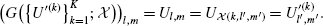(6)

As will be discussed in Sect. 4.1, the SMF-term expression (5) under the variational Bayesian approximation induces low-rank sparsity in each partition. This means that partition-wise sparsity is also induced. Accordingly, partitioning, rearrangement, and factorization should be designed in the following manner. Suppose that we are given a required sparsity structure on a matrix (examples of possible side information that suggests particular sparsity structures are given in Sect. 2.3). We first partition the matrix, according to the required sparsity. Some partitions can be submatrices. We rearrange each of the submatrices on which we do not want to impose low-rank sparsity into a long vector (U(3) in the example in Fig. 1). We leave the other submatrices which we want to be low-rank (U(2)), the vectors (U(1) and U(4)) and the scalars (U(5)) as they are. Finally, we factorize each of the PR matrices to induce sparsity through the MIR mechanism.

Let us, for example, assume that row-wise sparsity is required. We first make the row-wise partition, i.e., separate $$U \in\mathbb {R}^{L \times M}$$ into L pieces of M-dimensional row vectors $$U'^{(l)} =\widetilde {\boldsymbol {u}}_{l}^{{\top }} \in\mathbb{R}^{1 \times M}$$. Then, we factorize each partition as U(l)=B (l) A (l)⊤ (see the top illustration in Fig. 2). Thus, we obtain the row-wise sparse term (2). Here, $$\mathcal{X}(k,1, m') = (k, m')$$ makes the following connection between Eqs. (2) and (5): $$\gamma_{l}^{e} = B^{(k)} \in\mathbb{R}, \widetilde{\boldsymbol {d}}_{l} = A^{(k)} \in\mathbb{R}^{M \times1} \mbox{ for } k=l$$. Similarly, requiring column-wise and element-wise sparsity leads to Eqs. (3) and (4), respectively (see the bottom two illustrations in Fig. 2). Table 1 summarizes how to design these SMF terms, where vec-order(k)=(1+((k−1) mod L),⌈k/L⌉) goes along the columns one after another in the same way as the vec operator forming a vector by stacking the columns of a matrix (in other words, (U(1),…,U(K))=vec(U)).

We define a sparse additive matrix factorization (SAMF) model as the sum of SMF terms (5):(7)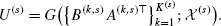(8)

In practice, SMF terms should be designed based on side information. Suppose that $$V \in\mathbb{R}^{L \times M}$$ consists of M samples of L-dimensional sensor outputs. In robust PCA (Candès et al. 2011; Ding et al. 2011; Babacan et al. 2012), an element-wise sparse term is added to the low-rank term, which is expected to be the clean signal, when sensor outputs are expected to contain spiky noise: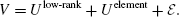(9)

Here, it can be said that the “expectation of spiky noise” is used as side information.

Similarly, if we suspect that some sensors are broken, and their outputs are unreliable over all M samples, we should prepare the row-wise sparse term to capture the expected row-wise noise, and try to keep the estimated clean signal U low-rank uncontaminated with the row-wise noise: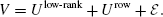If we know that some accidental disturbances occurred during the observation, but do not know their exact locations (i.e., which samples are affected), the column-wise sparse term can effectively capture these disturbances.

The SMF expression (5) enables us to use side information in a more flexible way. In Sect. 5.4, we show that our method can be applied to a foreground/background video separation problem, where moving objects (such as a person in Fig. 3) are considered to belong to the foreground. Previous approaches (Candès et al. 2011; Ding et al. 2011; Babacan et al. 2012) constructed the observation matrix V by stacking all pixels in each frame into each column (Fig. 4), and fitted it by the model (9). Here, the low-rank term and the element-wise sparse term are expected to capture the static background and the moving foreground, respectively. However, we can also rely on a natural assumption that a pixel segment having similar intensity values in an image tends to belong to the same object. Based on this side information, we adopt a segment-wise sparse term, where the PR matrix is constructed using a precomputed over-segmented image (Fig. 5). We will show in Sect. 5.4 that the segment-wise sparse term captures the foreground more accurately than the element-wise sparse term.

Let us summarize the parameters of the SAMF model (7) as follows: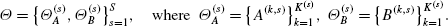As in the probabilistic MF (Salakhutdinov and Mnih 2008), we assume independent Gaussian noise and priors. Thus, the likelihood and the priors are written as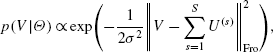(10)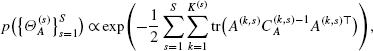(11)(12)

where ∥⋅∥Fro and tr(⋅) denote the Frobenius norm and the trace of a matrix, respectively. We assume that the prior covariances of A (k,s) and B (k,s) are diagonal and positive-definite: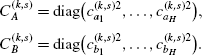Without loss of generality, we assume that the diagonal entries of $$C_{A}^{(k,s)} C_{B}^{(k,s)}$$ are arranged in the non-increasing order, i.e., $$c_{a_{h}}^{(k,s)} c_{b_{h}}^{(k,s)} \geq c_{a_{h'}}^{(k,s)} c_{b_{h'}}^{(k,s)}$$ for any pair h<h′.

### Variational Bayesian approximation

The Bayes posterior is written as(13)

where p(V)=〈p(V|Θ)〉 p(Θ) is the marginal likelihood. Here, 〈⋅〉 p denotes the expectation over the distribution p. Since the Bayes posterior (13) for matrix factorization is computationally intractable, the variational Bayesian (VB) approximation was proposed (Bishop 1999; Lim and Teh 2007; Ilin and Raiko 2010; Babacan et al. 2012).

Let r(Θ), or r for short, be a trial distribution. The following functional with respect to r is called the free energy:(14)

The first term is the Kullback-Leibler (KL) distance from the trial distribution to the Bayes posterior, and the second term is a constant. Therefore, minimizing the free energy (14) amounts to finding a distribution closest to the Bayes posterior in the sense of the KL distance. In the VB approximation, the free energy (14) is minimized over some restricted function space.

Following the standard VB procedure (Bishop 1999; Lim and Teh 2007; Babacan et al. 2012), we impose the following decomposability constraint on the posterior: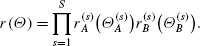(15)

Under this constraint, it is easy to show that the VB posterior minimizing the free energy (14) is written as(16)

where $$\mathcal{N}_{d}(\cdot; \boldsymbol {\mu }, \varSigma)$$ denotes the d-dimensional Gaussian distribution with mean μ and covariance Σ.

## Algorithm for SAMF

In this section, we first present a theorem that reduces a partial SAMF problem to the standard MF problem, which can be solved analytically. Then we derive an algorithm for the entire SAMF problem.

### Key theorem

Let us denote the mean of U (s), defined in Eq. (8), over the VB posterior by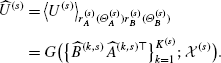(17)

Then we obtain the following theorem (its proof is given in Appendix A):

### Theorem 1

Given $$\{\widehat{U}^{(s')}\}_{s' \ne s}$$ and the noise variance σ 2, the VB posterior of $$(\varTheta_{A}^{(s)}, \varTheta_{B}^{(s)}) = \{A^{(k, s)}, B^{(k, s)}\} _{k=1}^{K^{(s)}}$$ coincides with the VB posterior of the following MF model: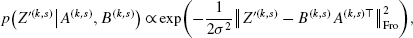(18)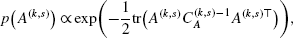(19)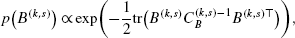(20)

for each k=1,…,K (s). Here, $$Z'^{(k,s)} \in\mathbb{R}^{L'^{(k,s)} \times M'^{(k,s)}}$$ is defined as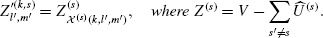(21)

The left formula in Eq. (21) relates the entries of $$Z^{(s)} \in\mathbb{R}^{L \times M}$$ to the entries of $$\{Z'^{(k, s)} \in\mathbb{R}^{L'^{(k, s)} \times M'^{(k, s)}} \}_{k=1}^{K^{(s)}}$$ by using the map $$\mathcal{X}^{(s)} : (k, l', m') \mapsto(l, m)$$ (see Eq. (6) and Fig. 1).

Theorem 1 states that a partial problem of SAMF—finding the posterior of (A (k,s),B (k,s)) for each k=1,…,K(s), given $$\{\widehat {U}^{(s')}\}_{s' \ne s}$$ and σ 2—can be solved in the same way as in the standard VBMF, to which the global analytic solution is available (Nakajima et al. 2013). Based on this theorem, we will propose a useful algorithm in the following subsections.

The noise variance σ 2 is also unknown in many applications. To estimate σ 2, we can use the following lemma (its proof is also included in Appendix A):

### Lemma 1

Given the VB posterior for $$\{\varTheta_{A}^{(s)}, \varTheta_{B}^{(s)}\}_{s=1}^{S}$$, the noise variance σ 2 minimizing the free energy (14) is given by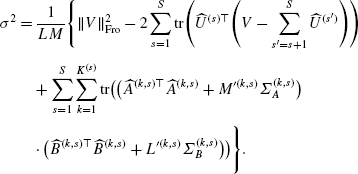(22)

### Partial analytic solution

Theorem 1 allows us to use the results given in Nakajima et al. (2013), which provide the global analytic solution for VBMF. Although the free energy of VBMF is also non-convex, Nakajima et al. (2013) showed that the minimizers can be written as a reweighted singular value decomposition. This allows one to solve the minimization problem separately for each singular component, which facilitated the analysis. By finding all stationary points and calculating the free energy on them, they successfully obtained an analytic-form of the global VBMF solution.

Combining Theorem 1 above and Theorems 3–5 in Nakajima et al. (2013), we obtain the following corollaries:

### Corollary 1

Assume that L(k,s)M(k,s) for all (k,s), and that $$\{\widehat{U}^{(s')}\}_{s' \ne s}$$ and the noise variance σ 2 are given. Let $$\gamma_{h}^{(k,s)}$$ (≥0) be the h-th largest singular value of Z(k,s), and let $$\boldsymbol {\omega }_{a_{h}}^{(k,s)}$$ and $$\boldsymbol {\omega }_{b_{h}}^{(k,s)}$$ be the associated right and left singular vectors: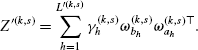(23)

Let $$\widehat{\gamma}_{h}^{(k,s)\mathrm{second}}$$ be the second largest real solution of the following quartic equation with respect to t:(24)

where the coefficients are defined byLet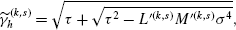(25)

where

$$\tau= \frac{(L'^{(k,s)}+M'^{(k,s)})\sigma^2}{2}+\frac{\sigma^4}{2 c_{a_h}^{(k,s)2} c_{b_h}^{(k,s)2}}.$$

Then, the global VB solution can be expressed as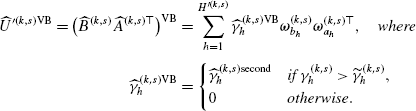(26)

### Corollary 2

Assume that L(k,s)M(k,s) for all (k,s). Given $$\{\widehat{U}^{(s')}\}_{s' \ne s}$$ and the noise variance σ 2, the global empirical VB solution (where the hyperparameters $$\{C_{A}^{(k,s)}, C_{B}^{(k,s)}\}$$ are also estimated from observation) is given by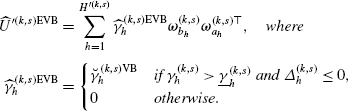(27)

Here,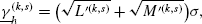(28)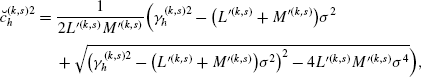(29)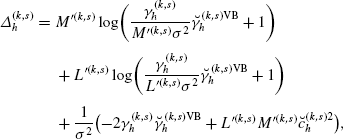(30)

and $$\breve{\gamma}_{h}^{(k,s)\mathrm{VB}}$$ is the VB solution for $$c_{a_{h}}^{(k,s)} c_{b_{h}}^{(k,s)}=\breve{c}_{h}^{(k,s)}$$.

### Corollary 3

Assume that L(k,s)M(k,s) for all (k,s). Given $$\{\widehat{U}^{(s')}\}_{s' \ne s}$$ and the noise variance σ 2, the VB posteriors are given by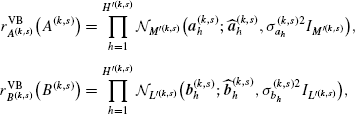where, for $$\widehat{\gamma}_{h}^{(k,s)\mathrm{VB}}$$ being the solution given by Corollary 1,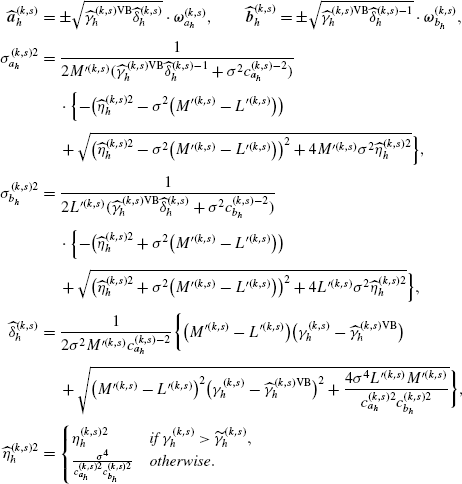Note that the corollaries above assume that L(k,s)M(k,s) for all (k,s). However, we can easily obtain the result for the case when L(k,s)>M(k,s) by considering the transpose $$\widehat{U}'^{(k, s){\top }}$$ of the solution. Also, we can always take the mapping $$\mathcal{X}^{(s)}$$ so that L(k,s)M(k,s) holds for all (k,s) without any practical restriction. This eases the implementation of the algorithm.

When σ 2 is known, Corollaries 1 and 2 provide the global analytic solution of the partial problem, where the variables on which $$\{\widehat{U}^{(s')}\}_{s' \ne s}$$ depends are fixed. Note that they give the global analytic solution for single-term (S=1) SAMF.

### Mean update algorithm

Using Corollaries 1–3 and Lemma 1, we propose an algorithm for SAMF, which we call the mean update (MU) algorithm. We describe its pseudo-code in Algorithm 1, where $$0_{(d_{1}, d_{2})}$$ denotes the d 1×d 2 matrix with all entries equal to zero. Note that, under the empirical Bayesian framework, all unknown parameters are estimated from observation, which allows inference without manual parameter tuning.

The MU algorithm is similar in spirit to the backfitting algorithm (Hastie and Tibshirani 1986; D’Souza et al. 2004), where each additive term is updated to fit a dummy target. In the MU algorithm, Z (s) defined in Eq. (21) corresponds to the dummy target in the backfitting algorithm. Although each of the corollaries and the lemma above guarantee the global optimality for each step, the MU algorithm does not generally guarantee the simultaneous global optimality over the entire parameter space. Nevertheless, experimental results in Sect. 5 show that the MU algorithm performs very well in practice.

When Corollaries 1 or 2 is applied in Step 3 of Algorithm 1, a singular value decomposition (23) of Z(k,s), defined in Eq.(21), is required. However, for many practical SMF terms, including the row-wise, the column-wise, and the element-wise terms as well as the segment-wise term (which will be defined in Sect. 5.4), $$Z'^{(k, s)} \in\mathbb{R}^{L'^{(k, s)} \times M'^{(k, s)}}$$ is a vector or scalar, i.e., L(k,s)=1 or M(k,s)=1. In such cases, the singular value and the singular vectors are given simply by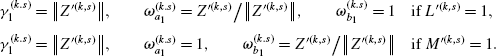## Discussion

In this section, we first relate MIR to ARD. Then, we introduce the standard VB iteration for SAMF, which is used as a baseline in the experiments. After that, we discuss related previous work, and the limitation of the current work.

### Relation between MIR and ARD

The MIR effect (Nakajima and Sugiyama 2011) induced by factorization actually has a close connection to the automatic relevance determination (ARD) effect (Neal 1996). Assume C A =I H , where I d denotes the d-dimensional identity matrix, in the plain MF model (18)–(20) (here we omit the suffixes k and s for brevity), and consider the following transformation: $$BA^{{\top }}\mapsto U \in\mathbb {R}^{L \times M}$$. Then, the likelihood (18) and the prior (19) on A are rewritten as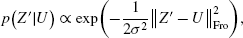(31)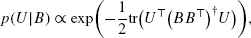(32)

where † denotes the Moore-Penrose generalized inverse of a matrix. The prior (20) on B is kept unchanged. p(U|B) in Eq. (32) is so-called the ARD prior with the covariance hyperparameter $$B B^{{\top }}\in \mathbb{R}^{L \times L}$$. It is known that this induces the ARD effect, i.e., the empirical Bayesian procedure where the prior covariance hyperparameter BB is also estimated from observation induces strong regularization and sparsity (Neal 1996); see also Efron and Morris (1973) for a simple Gaussian case.

In the current context, Eq. (32) induces low-rank sparsity on U if no restriction on BB is imposed. Similarly, we can show that $$(\gamma^{e}_{l})^{2}$$ in Eq. (2), $$(\gamma^{d}_{m})^{2}$$ in Eq. (3), and $$E_{l, m}^{2}$$ in Eq. (4) act as prior variances shared by the entries in $$\widetilde{\boldsymbol {u}}_{l} \in\mathbb{R}^{M}$$, $$\boldsymbol {u}_{m}\in\mathbb{R}^{L}$$, and $$U_{l, m} \in\mathbb{R}$$, respectively. This explains the mechanism how the factorization forms in Eqs. (2)–(4) induce row-wise, column-wise, and element-wise sparsity, respectively.

When we employ the SMF-term expression (5), MIR occurs in each partition. Therefore, partition-wise sparsity and low-rank sparsity in each partition is observed. Corollaries 1 and 2 theoretically support this fact: Small singular values are discarded by thresholding in Eqs. (26) and (27).

### Standard VB iteration

Following the standard procedure for the VB approximation (Bishop 1999; Lim and Teh 2007; Babacan et al. 2012), we can derive the following algorithm, which we call the standard VB iteration:(33)(34)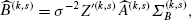(35)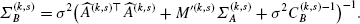(36)

Iterating Eqs. (33)–(36) for each (k,s) in turn until convergence gives a local minimum of the free energy (14).

In the empirical Bayesian scenario, the hyperparameters $$\{C_{A}^{(k,s)}, C_{B}^{(k,s)}\} _{k=1,}^{K^{(s)}}{}_{s=1}^{S}$$ are also estimated from observations. The following update rules give a local minimum of the free energy: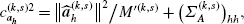(37)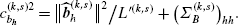(38)

When the noise variance σ 2 is unknown, it is estimated by Eq. (22) in each iteration.

The standard VB iteration is computationally efficient since only a single parameter in $$\{\widehat{A}^{(k, s)},\varSigma_{A}^{(k, s)},\widehat{B}^{(k, s)},\varSigma_{B}^{(k, s)}, c_{a_{h}}^{(k,s)2},c_{b_{h}}^{(k,s)2}\}_{k=1,}^{K^{(s)}}{}_{s=1}^{S}$$ is updated in each step. However, it is known that the standard VB iteration is prone to suffer from the local minima problem (Nakajima et al. 2013). On the other hand, although the MU algorithm also does not guarantee the global optimality as a whole, it simultaneously gives the global optimal solution for the set $$\{\widehat{A}^{(k, s)},\varSigma_{A}^{(k, s)},\widehat{B}^{(k, s)},\varSigma_{B}^{(k, s)}, c_{a_{h}}^{(k,s)2},c_{b_{h}}^{(k,s)2}\}_{k=1}^{K^{(s)}}$$ for each s in each step. In Sect. 5, we will experimentally show that the MU algorithm tends to give a better solution (i.e., with a smaller free energy) than the standard VB iteration.

### Related work

As widely known, traditional PCA is sensitive to outliers in data and generally fails in their presence. Robust PCA (Candès et al. 2011) was developed to cope with large outliers that are not modeled within the traditional PCA. Unlike methods based on robust statistics (Huber and Ronchetti 2009; Fischler and Bolles 1981; De la Torre and Black 2003; Ke and Kanade 2005; Gao 2008; Luttinen et al. 2009; Lakshminarayanan et al. 2011), Candès et al. (2011) explicitly modeled the spiky noise with an additional element-wise sparse term (see Eq. (9)). This model can also be applied to applications where the task is to estimate the element-wise sparse term itself (as opposed to discarding it as noise). A typical such application is foreground/background video separation (Fig. 3).

The original formulation of robust PCA is non-Bayesian, and the sparsity is induced by the 1-norm regularization. Although its solution can be efficiently obtained via the augmented Lagrange multiplier (ALM) method (Lin et al. 2009), there are unknown algorithmic parameters that should be carefully tuned to obtain its best performance. Employing a Bayesian formulation addresses this issue: A sampling-based method (Ding et al. 2011) and a VB method (Babacan et al. 2012) were proposed, where all unknown parameters are estimated from the observation.

Babacan et al. (2012) conducted an extensive experimental comparison between their VB method, called a VB robust PCA, and other methods. They reported that the ALM method (Lin et al. 2009) requires careful tuning of its algorithmic parameters, and the Bayesian sampling method (Ding et al. 2011) has high computational complexity that can be prohibitive in large-scale applications. Compared to these methods, the VB robust PCA is favorable both in terms of computational complexity and estimation performance.

Our SAMF framework contains the robust PCA model as a special case where the observed matrix is modeled as the sum of a low-rank and an element-wise sparse terms. The VB algorithm used in Babacan et al. (2012) is the same as the standard VB iteration introduced in Sect. 4.2, except a slight difference in the hyperprior setting. Accordingly, our proposal in this paper is an extension of the VB robust PCA in two ways—more variation in sparsity with different types of factorization and higher accuracy with the MU algorithm. In Sect. 5, we experimentally show advantages of these extensions. In our experiment, we use a SAMF counterpart of the VB robust PCA, named ‘LE’-SAMF in Sect. 5.1, with the standard VB iteration as a baseline method for comparison.

Group LASSO (Yuan and Lin 2006) also provides a framework for arbitrary sparsity design, where the sparsity is induced by the 1-regularization. Although the convexity of the group LASSO problem is attractive, it typically requires careful tuning of regularization parameters, as the ALM method for robust PCA. On the other hand, group-sparsity is induced by model-induced regularization in SAMF, and all unknown parameters can be estimated, based on the Bayesian framework.

Another typical application of MF is collaborative filtering, where the observed matrix has missing entries. Fitting the observed entries with a low-rank matrix enables us to predict the missing entries. Convex optimization methods with the trace-norm penalty (i.e., singular values are regularized by the 1-penalty) have been extensively studied (Srebro et al. 2005; Rennie and Srebro 2005; Cai et al. 2010; Ji and Ye 2009; Tomioka et al. 2010).

Bayesian approaches to MF have also been actively explored. A maximum a posteriori (MAP) estimation, which computes the mode of the posterior distributions, was shown to be equivalent to the 1-MF when Gaussian priors are imposed on factorized matrices (Srebro et al. 2005). Salakhutdinov and Mnih (2008) applied the Markov chain Monte Carlo method to MF for the fully-Bayesian treatment. The VB approximation (Attias 1999; Bishop 2006) has also been applied to MF (Bishop 1999; Lim and Teh 2007; Ilin and Raiko 2010), and it was shown to perform well in experiments. Its theoretical properties, including the model-induced regularization, have been investigated in Nakajima and Sugiyama (2011).

### Limitations of SAMF and MU algorithm

Here, we note the limitations of SAMF and the MU algorithm. First, in the current formulation, each SMF term is not allowed to have overlapping groups. This excludes important applications, e.g., simultaneous feature and sample selection problems (Jacob et al. 2009). Second, the MU algorithm cannot be applied when the observed matrix has missing entries, although SAMF itself still works with the standard VB iteration. This is because the global analytic solution, on which the MU algorithm relies, holds only for the fully-observed case. Third, we assume the Gaussian distribution for the dense noise ($$\mathcal{E}$$ in Eq. (7)), which may not be appropriate for, e.g., binary observations. Variational techniques for non-conjugate likelihoods, such as the one used in Seeger and Bouchard (2012), are required to extend SAMF to more general noise distributions. Fourth, we rely on the VB inference so far, and have not known if the fully-Bayesian treatment with additional hyperpriors can improve the performance. Overcoming some of the limitations described above is a promising future work.

## Experimental results

In this section, we first experimentally compare the performance of the MU algorithm and the standard VB iteration. Then, we test the model selection ability of SAMF, based on the free energy comparison. After that, we demonstrate the usefulness of the flexibility of SAMF on benchmark datasets and in a real-world application.

### Mean update vs. standard VB

We compare the algorithms under the following model: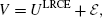where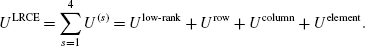(39)

Here, ‘LRCE’ stands for the sum of the Low-rank, Row-wise, Column-wise, and Element-wise terms, each of which is defined in Eqs. (1)–(4). We call this model ‘LRCE’-SAMF. As explained in Sect. 2.3, ‘LRCE’-SAMF may be used to separate the clean signal U low-rank from a possible row-wise sparse component (constantly broken sensors), a column-wise sparse component (accidental disturbances affecting all sensors), and an element-wise sparse component (randomly distributed spiky noise). We also evaluate ‘LCE’-SAMF, ‘LRE’-SAMF, and ‘LE’-SAMF, which can be regarded as generalizations of robust PCA (Candès et al. 2011; Ding et al. 2011; Babacan et al. 2012). Note that ‘LE’-SAMF corresponds to an SAMF counterpart of robust PCA.

First, we conducted an experiment with artificial data. We assume the empirical VB scenario with unknown noise variance, i.e., the hyperparameters $$\{C_{A}^{(k,s)}, C_{B}^{(k,s)}\}_{k=1,}^{K^{(s)}}{}_{s=1}^{S}$$ and the noise variance σ 2 are also estimated from observations. We use the full-rank model (H=min(L,M)) for the low-rank term U low-rank, and expect the MIR effect to find the true rank of U low-rank, as well as the non-zero entries in U row,U column, and U element.

We created an artificial dataset with the data matrix size L=40 and M=100, and the rank H =10 for a true low-rank matrix U low-rank∗=B A ∗⊤. Each entry in $$A^{*} \in\mathbb{R}^{M \times H^{*}}$$ and $$B^{*} \in\mathbb {R}^{L \times H^{*}}$$ was drawn from $$\mathcal{N}_{1}(0, 1)$$. A true row-wise (column-wise) part U row∗ (U column∗) was created by first randomly selecting ρL rows (ρM columns) for ρ=0.05, and then adding a noise subject to $$\mathcal{N}_{M}(\boldsymbol {0}, \zeta I_{M})$$ ($$\mathcal{N}_{L}(\boldsymbol {0}, \zeta I_{L})$$) for ζ=100 to each of the selected rows (columns). A true element-wise part U element∗ was similarly created by first selecting ρLM entries, and then adding a noise subject to $$\mathcal{N}_{1}(0, \zeta)$$ to each of the selected entries. Finally, an observed matrix V was created by adding a noise subject to $$\mathcal{N}_{1}(0, 1)$$ to each entry of the sum U LRCE∗ of the above four true matrices.

It is known that the standard VB iteration (reviewed in Sect. 4.2) is sensitive to initialization (Nakajima et al. 2013). We set the initial values in the following way: The mean parameters $$\{\widehat{A}^{(k, s)}, \widehat{B}^{(k, s)}\} _{k=1,}^{K^{(s)}}{}_{s=1}^{S}$$ were randomly created so that each entry follows $$\mathcal{N}_{1}(0, 1)$$. The covariances $$\{\varSigma_{A}^{(k, s)}, \varSigma_{B}^{(k, s)}\} _{k=1,}^{K^{(s)}}{}_{s=1}^{S}$$ and the hyperparameters $$\{C_{A}^{(k, s)}, C_{B}^{(k, s)}\} _{k=1,}^{K^{(s)}}{}_{s=1}^{S}$$ were set to be identity. The initial noise variance was set to σ 2=1. Note that we rescaled V so that $$\|V\|_{\mathrm{Fro}}^{2} / (LM)=1$$, before starting iteration. We ran the standard VB algorithm 10 times, starting from different initial points, and each trial is plotted by a solid line (labeled as ‘Standard(iniRan)’) in Fig. 6.

Initialization for the MU algorithm (described in Algorithm 1) is simple: We just set $$\widehat{U}^{(s)} = 0_{(L, M)}$$ for s=1,…,S, and σ 2=1. Initialization of all other variables is not needed. Furthermore, we empirically observed that the initial value for σ 2 does not affect the result much, unless it is too small. Note that, in the MU algorithm, initializing σ 2 to a large value is not harmful, because it is set to an adequate value after the first iteration with the mean parameters kept $$\widehat{U}^{(s)} = 0_{(L, M)}$$. The result with the MU algorithm is plotted by the dashed line in Fig. 6.

Figures 6(a)–6(c) show the free energy, the computation time, and the estimated rank, respectively, over iterations, and Fig. 6(d) shows the reconstruction errors after 250 iterations. The reconstruction errors consist of the overall error $$\|\widehat{U}^{\mathrm{LRCE}} - U^{\mathrm {LRCE*}} \|_{\mathrm{Fro}} / (LM)$$, and the four component-wise errors $$\|\widehat{U}^{(s)} - U^{(s)*} \| _{\mathrm{Fro}} / (LM)$$. The graphs show that the MU algorithm, whose iteration is computationally slightly more expensive than the standard VB iteration, immediately converges to a local minimum with the free energy substantially lower than the standard VB iteration. The estimated rank agrees with the true rank $$\widehat{H} = H^{*} = 10$$, while all 10 trials of the standard VB iteration failed to estimate the true rank. It is also observed that the MU algorithm well reconstructs each of the four terms.

We can slightly improve the performance of the standard VB iteration by adopting different initialization schemes. The line labeled as ‘Standard(iniML)’ in Fig. 6 indicates the maximum likelihood (ML) initialization, i.e, $$(\widehat{\boldsymbol {a}}_{h}^{(k, s)}, \widehat{\boldsymbol {b}}_{h}^{(k, s)}) = (\gamma _{h}^{(k, s) 1/2}\boldsymbol {\omega }_{a_{h}}^{(k, s)}, \gamma_{h}^{(k, s) 1/2}\boldsymbol {\omega }_{b_{h}}^{(k, s)})$$. Here, $$\gamma_{h}^{(k, s)}$$ is the h-th largest singular value of the (k,s)-th PR matrix V(k,s) of V (such that $$V'^{(k,s)}_{l', m'} = V_{\mathcal{X}^{(s)}(k, l', m')}$$), and $$\boldsymbol {\omega }_{a_{h}}^{(k, s)}$$ and $$\boldsymbol {\omega }_{b_{h}}^{(k, s)}$$ are the associated right and left singular vectors. Also, we empirically found that starting from small σ 2 alleviates the local minima problem. The line labeled as ‘Standard(iniMLSS)’ indicates the ML initialization with σ 2=0.0001. We can see that this scheme successfully recovered the true rank. However, the free energy and the reconstruction error are still substantially worse than the MU algorithm.

Figure 7 shows results with ‘LE’-SAMF when L=100, M=300, H =20, and ρ=0.1. We see that the MU algorithm compares favorably with the standard VB iteration. We have also tested various SAMF models including ‘LCE’-SAMF, ‘LRE’-SAMF, and ‘LE’-SAMF under different settings for L, M, H , and ρ, and empirically found that the MU algorithm generally gives a better solution with lower free energy and smaller reconstruction errors than the standard VB iteration.

Next, we conducted experiments with benchmark data. Since we do not know the true model of these data, we only focus on the achieved free energy, which directly indicates the approximation accuracy to the Bayes posterior (see Sect. 2.4). To this end, we simply fitted SAMF models to benchmark datasets by the MU algorithm and the standard VB iteration, and plotted the obtained free energy.

Figure 8 shows the free energy after convergence in ‘LRCE’-SAMF, ‘LCE’-SAMF, ‘LRE’-SAMF, and ‘LE’-SAMF on several datasets from the UCI repository (Asuncion and Newman 2007). For better comparison, a constant is added to the obtained free energy, so that the value of the MU algorithm is zero. We can see a clear advantage of the MU algorithm over the standard VB iteration.

Since the experiments in this subsection have shown the superiority of the MU algorithm over the standard VB iteration, the experiments in the following subsections are conducted only with the MU algorithm.

### Model selection ability

We tested the model selection ability of SAMF, by checking if the ‘LE’-SAMF, ‘LC’-SAMF, and ‘LR’-SAMF models give the lowest free energy for the artificial data created from the corresponding models, respectively.

We created artificial(‘LE’) data from a true ‘LE’-SAMF with L=150, M=200, H =20, ρ=0.1, and ζ=100. Likewise, we created artificial(‘LC’) and artificial(‘LR’) data from true ‘LC’-SAMF and ‘LR’-SAMF models, respectively. Then, we applied ‘LE’-SAMF, ‘LC’-SAMF, and ‘LR’-SAMF models to those artificial data, and plotted the obtained free energies in Fig. 9(a). If the model selection is successful, ‘LE’-SAMF should result in the lowest free energy than the other two models on the artificial(‘LE’) data. The same applies to ‘LC’-SAMF and ‘LR’-SAMF, respectively.

We see in Fig. 9(a) that the model selection is successful on the artificial(‘LE’) data and the artificial(‘LR’) data, but not on the artificial(‘LC’) data. In our investigation, we observed that SAMF sometimes mixes up the column-wise term with the low-rank term. We expect that a column-wise noise should be captured by the column-wise term. However, it can also be captured by either of the low-rank and the element-wise terms at the expense of a small loss of degrees of freedom (i.e., the problem is nearly ill-posed). The Bayesian regularization should choose the column-wise term, because it uses the lowest degrees of freedom to capture the column-wise noise. We suspect that the difference in regularization between the low-rank and the column-wise terms is too small for stable model selection against the disturbance by random noise and the existence of local minima. We also conducted the same experiment, of which the result is shown in Fig. 9(b), with stronger sparse noise with ζ=100LM. In this case, the model selection is successful on all three artificial data. Further investigation on handling these nearly ill-posed cases is left as future work.

### Robustness against simulated sparse noise

Here, we experimentally show the usefulness of the SAMF extension beyond the robust PCA with simulated sparse noise.

Datasets from the UCI repository consist of M samples with L dimensions. We simulated sparse noise that contaminates a small number of measurements over the samples. We also simulated some accidents that cause simultaneous contamination in all the measurements of a small number of samples. As explained in Sect. 2.3, the SAMF model can capture the former type of noise by the element-wise sparse term, and the latter type of noise by the column-wise sparse term.

We created semi-artificial data in the following procedure. We first rescaled the benchmark data V org so that $$\|V^{\textrm{org}}\|_{\mathrm{Fro}}^{2} / (LM)=1$$. Then, artificial true sparse noise components, U column∗ and U element∗, were created in the same way as in Sect. 5.1 with ρ=0.05, and added to V org, i.e.,Since we do not know the true model of the original benchmark data, we focus on robustness against the simulated sparse noise. For the column-wise sparse term, we evaluate the following value: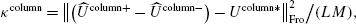where $$\widehat{U}^{\textrm{column}+}$$ is the column-wise sparse term estimated from the simulated data V sim, and $$\widehat{U}^{\textrm{column}-}$$ is the column-wise sparse term estimated from the original data V org. If a SAMF model perfectly captures the simulated true column-wise sparse noise U column∗ with its column-wise sparse term, then κ column=0 because the estimated column-wise sparse term is increased by the simulated noise, i.e., $$\widehat{U}^{\textrm{column}+} - \widehat{U}^{\textrm{column}-} = U^{\textrm{column}*}$$. Therefore, smaller κ column is expected to indicate higher robustness of the model against the sparse noise. κ element is calculated in the same way, and κ low-rank and κ row-wise are calculated without the simulated noise term, i.e., U low-rank∗=U row-wise∗=0(L,M).

Figure 10 shows the values of κ averaged over 10 trials with randomly created sparse noise. As expected, the SAMF models (‘LRCE’-SAMF and ‘LCE’-SAMF) having the column-wise sparse term are more reliable than the others (‘LRE’-SAMF and ‘LE’-SAMF).

### Real-world application

Finally, we demonstrate the usefulness of the flexibility of SAMF in a foreground (FG)/background (BG) video separation problem (Fig. 3). Candès et al. (2011) formed the observed matrix V by stacking all pixels in each frame into each column (Fig. 4), and applied robust PCA (with ‘LE’-terms)—the low-rank term captures the static BG and the element-wise (or pixel-wise) term captures the moving FG, e.g., people walking through. As discussed in Sect. 4.3, SAMF is an extension of the VB robust PCA (Babacan et al. 2012), which is the current state-of-the-art. We use ‘LE’-SAMF,which is conceptually the same as the VB robust PCA, as a baseline method for comparison.

The SAMF framework enables a fine-tuned design for the FG term. Assuming that pixels in an image segment with similar intensity values tend to share the same label (i.e., FG or BG), we formed a segment-wise sparse SMF term: U(k) for each k is a column vector consisting of all pixels in each segment. We produced an over-segmented image from each frame by using the efficient graph-based segmentation (EGS) algorithm (Felzenszwalb and Huttenlocher 2004), and substituted the segment-wise sparse term for the FG term (see Fig. 5):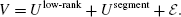We call this method segmentation-based SAMF (sSAMF). Note that EGS is computationally very efficient: It takes less than 0.05 sec on a usual laptop to segment a 192×144 grey image. EGS has several tuning parameters, and the obtained segmentation is sensitive to some of them. However, we confirmed that sSAMF performs similarly with visually different segmentations obtained over a wide range of tuning parameters (see detailed information below on the segmentation algorithm). Therefore, careful parameter tuning of EGS is not necessary for our purpose.

We compared sSAMF with ‘LE’-SAMF on the ‘WalkByShop1front’ video from the Caviar dataset.Footnote 1 Thanks to the Bayesian framework, all unknown parameters (except the ones for segmentation) are estimated automatically with no manual parameter tuning. For both models (‘LE’-SAMF and sSAMF), we used the MU algorithm, which has been shown in Sect. 5.1 to be practically more reliable than the standard VB iteration. The original video consists of 2360 frames, each of which is a color image with 384×288 pixels. We resized each image into 192×144 pixels, averaged over the color channels, and sub-sampled every 15 frames (the frame IDs are 0,15,30,…,2355). Thus, V is of the size of 27684 (pixels) × 158 (frames). We evaluated ‘LE’-SAMF and sSAMF on this video, and found that both models perform well (although ‘LE’-SAMF failed in a few frames).

To contrast the methods more clearly, we created a more difficult video by sub-sampling every 5 frames from 1501 to 2000 (the frame IDs are 1501,1506,…,1996 and V is of the size of 27684 (pixels) × 100 (frames)). Since more people walked through in this period, BG estimation is more challenging. The result is shown in Fig. 11.

Figure 11(a) shows an original frame. This is a difficult snap shot, because a person stayed at a same position for a while, which confuses separation. Figures 11(c) and 11(d) show the BG and the FG terms obtained by ‘LE’-SAMF, respectively. We can see that ‘LE’-SAMF failed to separate the person from BG (the person is partly captured in the BG term). On the other hand, Figs. 11(e) and 11(f) show the BG and the FG terms obtained by sSAMF based on the segmented image shown in Fig. 11(b). We can see that sSAMF successfully separated the person from BG in this difficult frame. A careful look at the legs of the person makes us understand how segmentation helps separation—the legs form a single segment (light blue colored) in Fig. 11(b), and the segment-wise sparse term (Fig. 11(f)) captured all pixels on the legs, while the pixel-wise sparse term (Fig. 11(d)) captured only a part of those pixels.

We observed that, in all frames of the difficult video, as well as the easier one, sSAMF gave good separation, while ‘LE’-SAMF failed in several frames (see movies provided as Online Resource).

For reference, we applied the convex optimization approach (Candès et al. 2011), which solves the minimization problem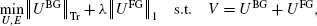where ∥⋅∥Tr and ∥⋅∥1 denote the trace norm and the 1-norm of a matrix, respectively, by the inexact ALM algorithm (Lin et al. 2009). Figure 12 shows the obtained BG and FG terms of the same frame as in Fig. 11 with λ=0.001,0.005,0.025. We see that the performance strongly depends on the parameter value of λ, and that sSAMF gives an almost identical result (bottom row in Fig. 11) to the best ALM result with λ=0.005 (middle row in Fig. 12) without any manual parameter tuning.

Below, we give detailed information on the segmentation algorithm, the computation time, and Online Resource.

### Segmentation algorithm

For the efficient graph-based segmentation (EGS) algorithm (Felzenszwalb and Huttenlocher 2004), we used the code publicly available from the authors’ homepage.Footnote 2 EGS has three tuning parameters: sigma, the smoothing parameter; k, the threshold parameter; minc, minimum segment size. Among them, k dominantly determines the typical size of segments (larger k leads to larger segments). To obtain over-segmented images for sSAMF in our experiment, we chose k=50, and the other parameters are set to sigma=0.5 and minc=20 as recommended by the authors. We also tested other parameter setting, and observed that FG/BG separation by sSAMF performed almost equally for 1≤k≤100, despite the visual variation of segmented images (see Fig. 13). Overall, we empirically observed that the performance of sSAMF is not very sensitive to the selection of segmented images, unless it is highly under-segmented.

### Computation time

The computation time for segmentation by EGS was less than 10 sec (for 100 frames). Forming the one-to-one map $$\mathcal{X}$$ took more than 80 sec (which is expected to be improved). In total, sSAMF took 600 sec on a Linux machine with Xeon X5570 (2.93 GHz), while ‘LE’-SAMF took 700 sec. This slight reduction in computation time comes from the reduction in the number K of partitions for the FG term, and hence the number of calculations of partial analytic solutions.

### Online resource

Online Resource consists of two movies that show the performance of ‘LE’-SAMF (a SAMF counterpart of robust PCA) and sSAMF over all frames of the easy video (10994_2013_5347_MOESM1_ESM.mpg) and the difficult video (10994_2013_5347_MOESM2_ESM.mpg). The format of both movies is exactly the same as Fig. 11, i.e., the top row shows an original frame and its segmentation, the middle row shows the BG and the FG terms obtained by ‘LE’-SAMF, and the bottom row shows the BG and the FG terms obtained by sSAMF.

## Conclusion

In this paper, we proposed a sparse additive matrix factorization (SAMF) model, which allows us to design various forms of factorization that induce various types of sparsity. We then proposed a variational Bayesian (VB) algorithm called the mean update (MU), which gives the global optimal solution for a large subset of parameters in each step. Through experiments, we showed that the MU algorithm compares favorably with the standard VB iteration. We also demonstrated the usefulness of the flexibility of SAMF in a real-world foreground/background video separation experiment, where image segmentation is used for automatically designing an SMF term.

Future work is to overcome the limitations discussed in Sect. 4.4. Analysis of convergence properties of the MU algorithm, and theoretical elucidation of the reason why the MU algorithm tends to give a better solution than the standard VB algorithm are also our important future work.

## References

• Asuncion, A., & Newman, D. J. (2007). UCI machine learning repository. http://www.ics.uci.edu/~mlearn/MLRepository.html.

• Attias, H. (1999). Inferring parameters and structure of latent variable models by variational Bayes. In Proceedings of the fifteenth conference on uncertainty in artificial intelligence (UAI-99), San Francisco, CA (pp. 21–30). San Mateo: Morgan Kaufmann.

• Babacan, S. D., Luessi, M., Molina, R., & Katsaggelos, A. K. (2012). Sparse Bayesian methods for low-rank matrix estimation. IEEE Transactions on Signal Processing, 60(8), 3964–3977.

• Bishop, C. M. (1999). Variational principal components. In Proc. of international conference on artificial neural networks (Vol. 1, pp. 509–514).

• Bishop, C. M. (2006). Pattern recognition and machine learning. New York: Springer.

• Cai, J. F., Candès, E. J., & Shen, Z. (2010). A singular value thresholding algorithm for matrix completion. SIAM Journal on Optimization, 20(4), 1956–1982.

• Candès, E. J., Li, X., Ma, Y., & Wright, J. (2011). Robust principal component analysis? Journal of the ACM, 58(3), 1–37.

• De la Torre, F., & Black, M. J. (2003). A framework for robust subspace learning. International Journal of Computer Vision, 54, 117–142.

• Ding, X., He, L., & Carin, L. (2011). Bayesian robust principal component analysis. IEEE Transactions on Image Processing, 20(12), 3419–3430.

• D’Souza, A., Vijayakumar, S., & Schaal, S. (2004). The Bayesian backfitting relevance vector machine. In Proceedings of the 21st international conference on machine learning.

• Efron, B., & Morris, C. (1973). Stein’s estimation rule and its competitors—an empirical Bayes approach. Journal of the American Statistical Association, 68, 117–130.

• Felzenszwalb, P. F., & Huttenlocher, D. P. (2004). Efficient graph-based image segmentation. International Journal of Computer Vision, 59(2), 167–181.

• Fischler, M. A., & Bolles, R. C. (1981). Random sample consensus: a paradigm for model fitting with applications to image analysis and automated cartography. Communications of the ACM, 24, 381–385.

• Gao, J. (2008). Robust l1 principal component analysis and its Bayesian variational inference. Neural Computation, 20, 555–578.

• Hastie, T., & Tibshirani, R. (1986). Generalized additive models. Statistical Science, 1(3), 297–318.

• Hotelling, H. (1933). Analysis of a complex of statistical variables into principal components. Journal of Educational Psychology, 24, 417–441.

• Huber, P. J., & Ronchetti, E. M. (2009). Robust statistics. New York: Wiley.

• Ilin, A., & Raiko, T. (2010). Practical approaches to principal component analysis in the presence of missing values. Journal of Machine Learning Research, 11, 1957–2000.

• Jacob, L., Obozinski, G., & Vert, J. P. (2009). Group Lasso with overlap and graph Lasso. In Proceedings of the 26th international conference on machine learning.

• Ji, S., & Ye, J. (2009). An accelerated gradient method for trace norm minimization. In Proceedings of international conference on machine learning (pp. 457–464).

• Ke, Q., & Kanade, T. (2005). Robust l1 norm factorization in the presence of outliers and missing data by alternative convex programming. In IEEE conference computer vision and pattern recognition.

• Lakshminarayanan, B., Bouchard, G., & Archambeau, C. (2011). Robust Bayesian matrix factorisation. In Proceedings of international conference on artificial intelligence and statistics (Vol. 15).

• Lim, Y. J., & Teh, T. W. (2007). Variational Bayesian approach to movie rating prediction. In Proceedings of KDD cup and workshop.

• Lin, Z., Chen, M., & Ma, Y. (2009). The augmented lagrange multiplier method for exact recovery of corrupted low-rank matrices. UIUC Technical Report UILU-ENG-09-2215.

• Luttinen, J., Ilin, A., & Karhunen, J. (2009). Bayesian robust PCA for incomplete data. In International conference on independent component analysis and signal separation.

• Nakajima, S., & Sugiyama, M. (2011). Theoretical analysis of Bayesian matrix factorization. Journal of Machine Learning Research, 12, 2579–2644.

• Nakajima, S., Sugiyama, M., & Babacan, S. D. (2012). Sparse additive matrix factorization for robust PCA and its generalization. In S. C. H. Hoi & W. Buntine (Eds.), Proceedings of fourth Asian conference on machine learning (pp. 301–316).

• Nakajima, S., Sugiyama, M., Babacan, S. D., & Tomioka, R. (2013). Global analytic solution of fully-observed variational Bayesian matrix factorization. Journal of Machine Learning Research, 14, 1–37.

• Neal, R. M. (1996). Bayesian learning for neural networks. Berlin: Springer.

• Raman, S., Fuchs, T. J., Wild, P. J., Dahl, E., & Roth, V. (2009). The Bayesian group-lasso for analyzing contingency tables. In Proceedings of international conference on machine learning (pp. 881–888).

• Rennie, J. D. M., & Srebro, N. (2005). Fast maximum margin matrix factorization for collaborative prediction. In Proceedings of the 22nd international conference on machine learning (pp. 713–719).

• Salakhutdinov, R., & Mnih, A. (2008). Bayesian probabilistic matrix factorization using Markov chain Monte Carlo. In International conference on machine learning.

• Seeger, M., & Bouchard, G. (2012). Fast variational Bayesian inference for non-conjugate matrix factorization models. In Proceedings of international conference on artificial intelligence and statistics, La Palma, Spain

• Srebro, N., Rennie, J., & Jaakkola, T. (2005). Maximum margin matrix factorization. In Advances in neural information processing systems (Vol. 17).

• Tomioka, R., Suzuki, T., Sugiyama, M., & Kashima, H. (2010). An efficient and general augmented Lagrangian algorithm for learning low-rank matrices. In Proceedings of international conference on machine learning.

• Watanabe, S. (2009). Algebraic geometry and statistical learning. Cambridge: Cambridge University Press.

• Yuan, M., & Lin, Y. (2006). Model selection and estimation in regression with grouped variables. Journal of the Royal Statistical Society B, 68(1), 49–67.

## Acknowledgements

Shinichi Nakajima and Masashi Sugiyama thank the support from Grant-in-Aid for Scientific Research on Innovative Areas: Prediction and Decision Making, 23120004. S. Derin Babacan was supported by a Beckman Postdoctoral Fellowship.

## Author information

Authors

### Corresponding author

Correspondence to Shinichi Nakajima.

Editors: Zhi-Hua Zhou, Wee Sun Lee, Steven Hoi, Wray Buntine, and Hiroshi Motoda.

## Electronic Supplementary Material

Below are the links to the electronic supplementary material.

(MPG 3.9 MB)

(MPG 2.5 MB)

## Appendix A: Proof of Theorem 1 and Lemma 1

### Appendix A: Proof of Theorem 1 and Lemma 1

First, we consider the single-term SAMF (S=1). In this case, the likelihood and the priors are written as follows:(40)(41)(42)

Let $$V'^{(k)} \in\mathbb{R}^{L'{(k)} \times M'{(k)}}$$ be the partitioned-and-rearranged (PR) observed matrix for k-th partition, i.e.,(43)

Since the map $$\mathcal{X}$$ is one-to-one, the following lemma holds:

### Lemma 2

Equation (40) can be factorized as follows:(44)

Next, we consider the general case when S≥1. Substituting Eqs. (10)–(12) and (16) into Eq. (14), we obtain the following lemma:

### Lemma 3

The free energy (14) for SAMF under the constraint (15) is given by(45)

Combining Lemmas 2 and 3, we have the following lemma:

### Lemma 4

Given $$\{\widehat{U}^{(s)}\}_{s' \ne s} = \{\{\widehat{B}^{(k, s')} \widehat{A}^{(k, s'){\top }} \}_{k=1}^{K^{(s')}}\}_{s' \ne s}$$, the free energy (14) for SAMF under the constraint (15) can be expressed as a function of $$\{\widehat{A}^{(k, s)}, \widehat{B}^{(k, s)}, \varSigma_{A}^{(k,s)}, \varSigma_{B}^{(k,s)} \}_{k=1}^{K^{(s)}}$$ as follows:where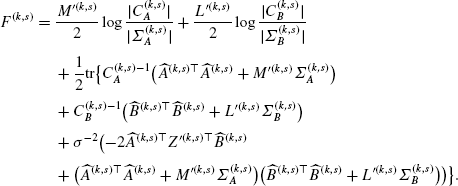(46)

The following proposition is known:

### Proposition 1

(Bishop 1999; Lim and Teh 2007): The VB posterior for the plain MF model(47)(48)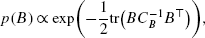(49)

is written as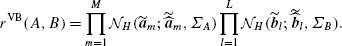(50)

The free energy is written as(51)

Now, we find that, given $$\{\widehat{U}^{(s)}\}_{s' \ne s} = \{\{\widehat{B}^{(k, s')} \widehat{A}^{(k, s'){\top }} \}_{k=1}^{K^{(s')}}\}_{s' \ne s}$$, Eqs. (16) and (46) for each (k,s) reduce to Eqs. (50) and (51), respectively, where V is replaced with Z(k,s). This completes the proof of Theorem 1.

Finally, we consider the noise variance σ 2 estimation. By assumption, we know all values of $$\{\{ \widehat{A}^{(k, s)}, \widehat{B}^{(k, s)}, \varSigma _{A}^{(k,s)}, \varSigma_{B}^{(k,s)} \}_{k=1}^{K^{(s)}}\}_{s=1}^{S}$$ that specify the VB posterior on $$\{\varTheta_{A}^{(s)}, \varTheta _{B}^{(s)}\}_{s=1}^{S}$$. $$\{\{ \varSigma_{A}^{(k,s)}, \varSigma_{B}^{(k,s)} \}_{k=1}^{K^{(s)}}\} _{s=1}^{S}$$ are positive-definite, because they are covariance matrices. Then, Eq. (45) goes to infinity either when σ 2→0 or when σ 2→∞. Furthermore, Eq. (45) is differentiable with respect to σ 2(>0). Consequently, any minimizer of Eq. (45) is necessarily a stationary point. By differentiating Eq. (45), we obtain Eq. (22) as a stationarity condition, which proves Lemma 1.

## Rights and permissions

Reprints and Permissions

Nakajima, S., Sugiyama, M. & Babacan, S.D. Variational Bayesian sparse additive matrix factorization. Mach Learn 92, 319–347 (2013). https://doi.org/10.1007/s10994-013-5347-6

• Accepted:

• Published:

• Issue Date:

• DOI: https://doi.org/10.1007/s10994-013-5347-6

### Keywords

• Variational Bayes
• Robust PCA
• Matrix factorization
• Sparsity
• Model-induced regularization